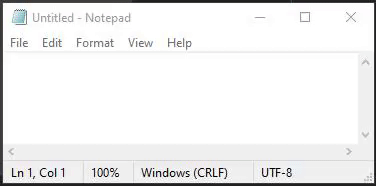# How do I make a TextLabel that counts the amount of letters in the current line of a textbox?

How do I make a TextLabel that counts the amount of letters in the current line of a textbox? (This is my first time making a topic on the devforums so sorry if I did anything wrong)

1 Like

You can use `string.len`

``````print(string.len(game.Workspace.Part.SurfaceGui.TextLabel.Text))
``````

1 Like

If you’re looking for letters specifically, you’d have to iterate through the string and count +1 to a variable each time a letter is found.

If you want the number of characters which includes alphanumeric and whitespaces then you can use `string.len`

If you want only letters you can use regex which would just be

``````local number_of_letters = 0
local func = string.gmatch(textBox.Text, "%a")
while func() ~= nil do
number_of_letters+=1
end
``````
1 Like

string.len returns a string / textLabel.Texts length.

Code example:

``````local textBox = -- path to text box

local lengthOfText = string.len(textBox.Text)

print(lengthOfText) -- Would print the letter of characters / letters etc.
``````

First, insert a local script inside your text box, then try this:

``````local textBox = script.Parent
local runService = game:GetService("RunService")

runService.Heartbeat:Connect(function(deltaTime)

local text = textBox.Text

print(#text)

end)
``````

And I’m not sure if this is want you want.

1 Like

Since nobody seems to understand what I mean,Something like this where it counts the number of letters in the current line.

Each line is separated invisibly by `\n`. To get each line individually you can use:

``````local stringLines = string.split(TextBox.Text, "\n");
-- stringLines = "This is the first line"
-- stringLines = "This is the second line"
...
``````
1 Like

This works but how do I find which line the user is on?

If you want to find a player from a string on a certain just loop them

``````local player;
for _, line in pairs(stringLines) do
if game:GetService("Players"):FindFirstChild(line) then
player = game:GetService("Players"):FindFirstChild(line);
break;
end
end
``````